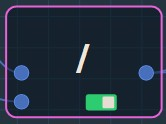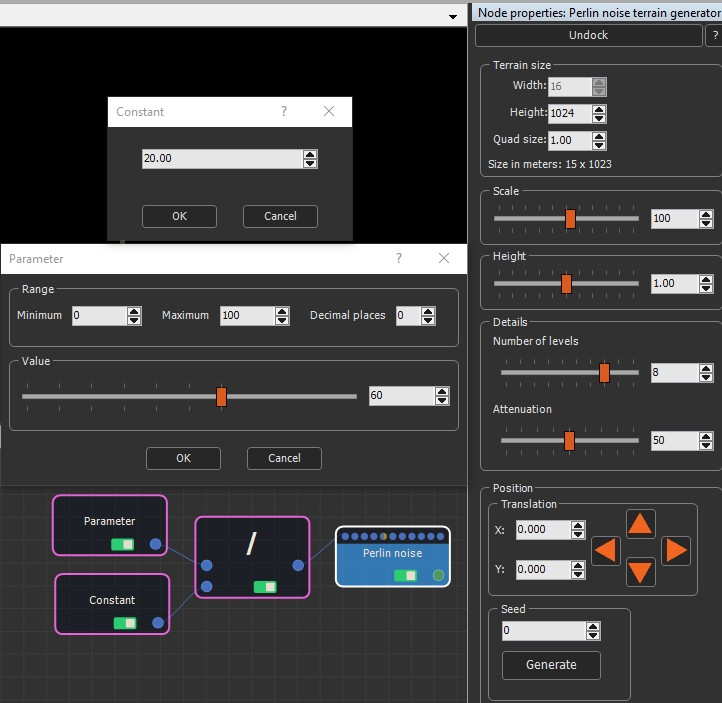The Divide node implements a division on two input numbers to generate an output number. It is an alternative to the Formula node, but allows you to code a formula as a graph rather than a formula.

It has two input connectors and one output connector and divides the first input value over the second input value.

To add a Divide node, select Edit in the main menu or right-click in the Graph Editor and select Create Node  > Calculation  > Divide.The Divide node has no parameters.